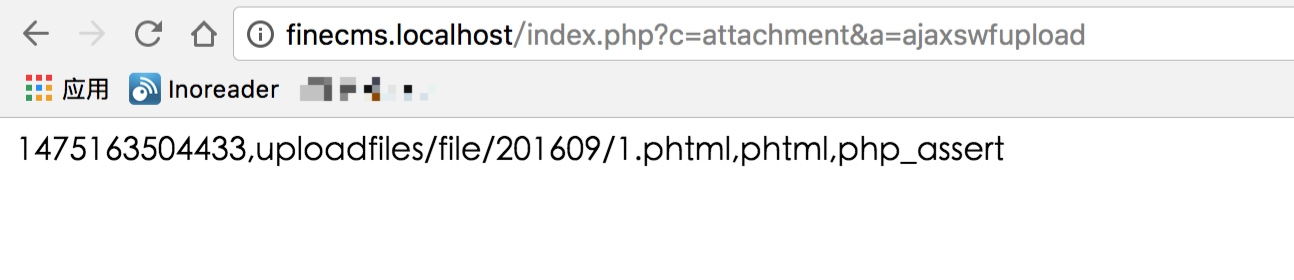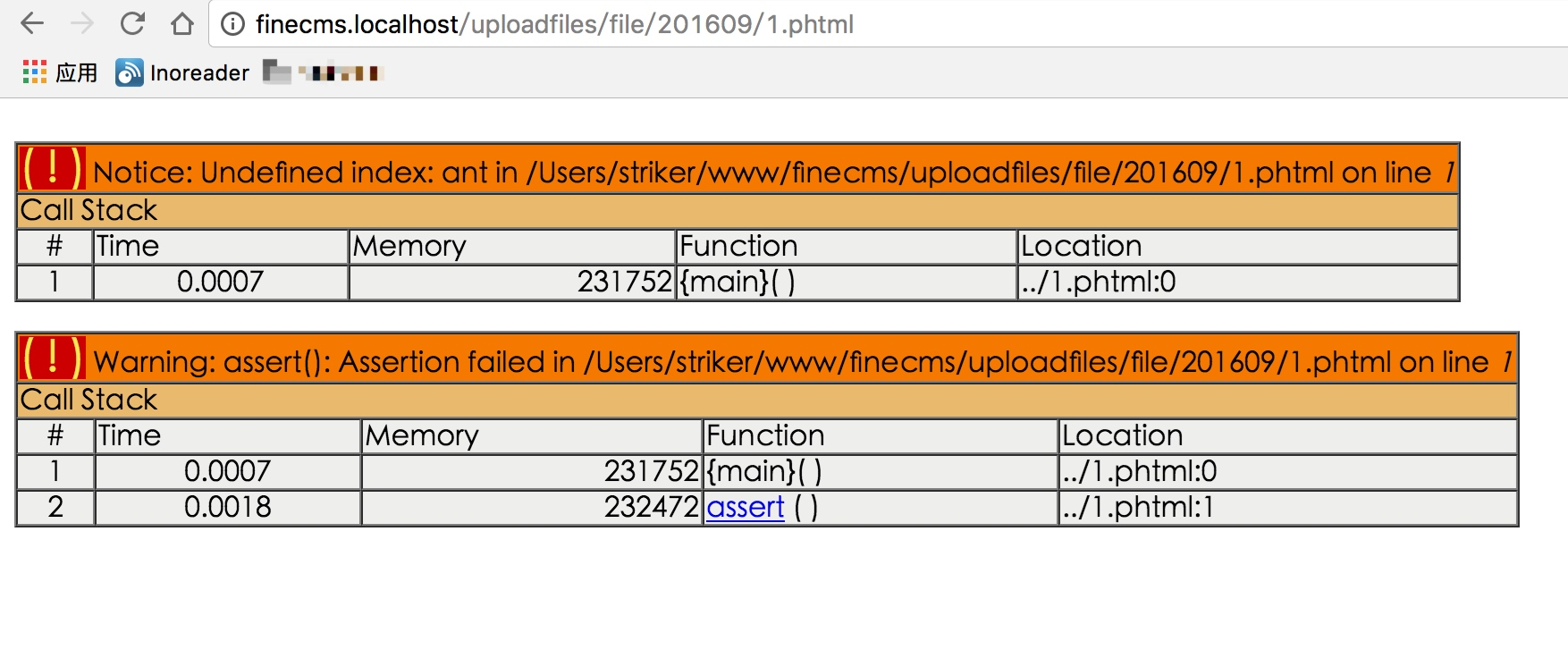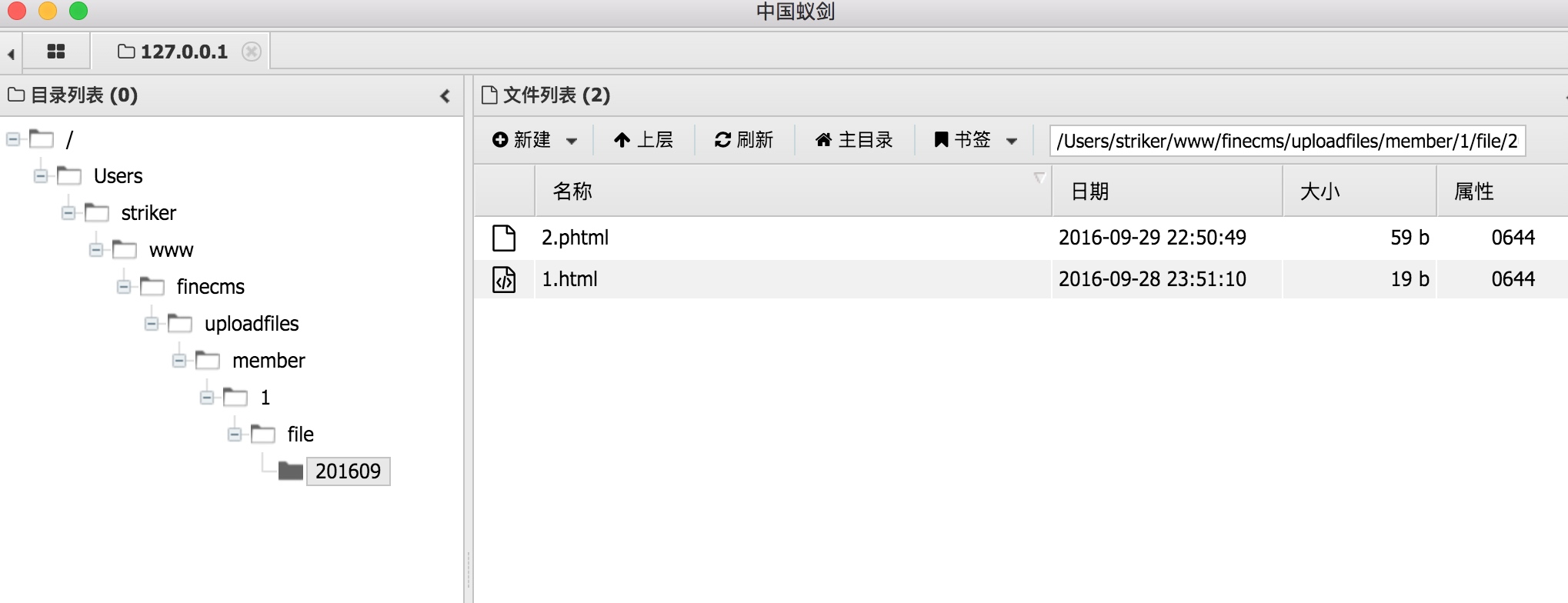Tuuu Nya's Blog

# FineCMS前台getshell

2016/09/30 ShareFineCMS企业网站管理系统（简称免费版或企业版）是一款基于PHP+MySql+CI框架开发的高效简洁的中小型内容管理系统，面向多终端包括Pc端网页和移动端网页，支持自定义内容模型和会员模型，并且可以自定义字段，可面向中小型站点提供重量级网站建设解决方案，适用于小型站点、企业级网站、新闻内容网站等。

## 漏洞概要

finecms某处过滤不严格，导致可上传任意脚本文件。

## 漏洞详情

``````/**
* Swf上传
*/
public function ajaxswfuploadAction() {
if (\$this->post('submit')) {
\$_type = explode(',', \$this->post('type'));
if (empty(\$_type)) {
exit('0,' . lang('att-6'));
}
\$size = (int)\$this->post('size');
if (empty(\$size)) {
exit('0,' . lang('att-5'));
}
\$data = \$this->upload('Filedata', \$_type, \$size, null, null, \$this->post('admin'), 'swf', null, \$this->post('document'));
if (\$data['result']) {
exit('0,' . \$data['result']);
}
//唯一ID,文件全路径,扩展名,文件名称
exit(time() . rand(0, 999) . ',' . \$data['path'] . ',' . \$data['ext'] . ',' . str_replace('|', '_', \$data['file']));
} else {
exit('0,' . lang('att-4'));
}
}
``````

``````\$ext = \$upload->fileext();
if (stripos(\$ext, 'php') !== FALSE
|| stripos(\$ext, 'asp') !== FALSE
|| stripos(\$ext, 'aspx') !== FALSE
) {
return array('result' => '文件格式被系统禁止');
``````

``````/**
* 取得文件扩展
*
* @return 扩展名
*/
public function fileext() {
return strtolower(trim(substr(strrchr(\$this->file_name['name'], '.'), 1, 10)));
}
``````

``````if (stripos(\$ext, 'php') !== FALSE
|| stripos(\$ext, 'asp') !== FALSE
|| stripos(\$ext, 'aspx') !== FALSE
) {
return array('result' => '文件格式被系统禁止');
``````

`stripos`函数从前往后查找`php``asp``aspx`如果查到了就直接return了。## 漏洞修复

``````if (stripos(\$ext, 'php') !== FALSE
|| stripos(\$ext, 'phtml') !== FALSE
|| stripos(\$ext, 'asp') !== FALSE
|| stripos(\$ext, 'aspx') !== FALSE
) {
return array('result' => '文件格式被系统禁止');
}
``````

CATALOG